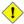# 9.5. Indirect References to Variables

Assume that the value of a variable is the name of a second variable. Is it somehow possible to retrieve the value of this second variable from the first one? For example, if a=letter_of_alphabet and letter_of_alphabet=z, can a reference to a return z? This can indeed be done, and it is called an indirect reference. It uses the unusual eval var1=\\$\$var2 notation.

Example 9-21. Indirect References

 ``` 1 #!/bin/bash 2 # Indirect variable referencing. 3  4 a=letter_of_alphabet 5 letter_of_alphabet=z 6  7 echo 8  9 # Direct reference. 10 echo "a = \$a" 11  12 # Indirect reference. 13 eval a=\\$\$a 14 echo "Now a = \$a" 15  16 echo 17  18  19 # Now, let's try changing the second order reference. 20  21 t=table_cell_3 22 table_cell_3=24 23 echo "\"table_cell_3\" = \$table_cell_3" 24 echo -n "dereferenced \"t\" = "; eval echo \\$\$t 25 # In this simple case, 26 # eval t=\\$\$t; echo "\"t\" = \$t" 27 # also works (why?). 28  29 echo 30  31 t=table_cell_3 32 NEW_VAL=387 33 table_cell_3=\$NEW_VAL 34 echo "Changing value of \"table_cell_3\" to \$NEW_VAL." 35 echo "\"table_cell_3\" now \$table_cell_3" 36 echo -n "dereferenced \"t\" now "; eval echo \\$\$t 37 # "eval" takes the two arguments "echo" and "\\$\$t" (set equal to \$table_cell_3) 38 echo 39  40 # (Thanks, S.C., for clearing up the above behavior.) 41  42  43 # Another method is the \${!t} notation, discussed in "Bash, version 2" section. 44 # See also example "ex78.sh". 45  46 exit 0```

Example 9-22. Passing an indirect reference to awk

 ``` 1 #!/bin/bash 2  3 # Another version of the "column totaler" script 4 # that adds up a specified column (of numbers) in the target file. 5 # This uses indirect references. 6  7 ARGS=2 8 E_WRONGARGS=65 9  10 if [ \$# -ne "\$ARGS" ] # Check for proper no. of command line args. 11 then 12  echo "Usage: `basename \$0` filename column-number" 13  exit \$E_WRONGARGS 14 fi 15  16 filename=\$1 17 column_number=\$2 18  19 #===== Same as original script, up to this point =====# 20  21  22 # A multi-line awk script is invoked by awk ' ..... ' 23  24  25 # Begin awk script. 26 # ------------------------------------------------ 27 awk " 28  29 { total += \\$\${column_number} # indirect reference 30 } 31 END { 32  print total 33  } 34  35  " "\$filename" 36 # ------------------------------------------------ 37 # End awk script. 38  39 # Indirect variable reference avoids the hassles 40 # of referencing a shell variable within the embedded awk script. 41 # Thanks, Stephane Chazelas. 42  43  44 exit 0```This method of indirect referencing is a bit tricky. If the second order variable changes its value, then the first order variable must be properly dereferenced (as in the above example). Fortunately, the \${!variable} notation introduced with version 2 of Bash (see Example 35-2) makes indirect referencing more intuitive.